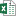VLOOKUP CHOOSE vs INDEX MATCH Performance Test - KING OF EXCEL

VLOOKUP CHOOSE vs INDEX MATCH Performance Test

Bottom Line: Which is faster? Let's compare the speeds of two popular formulas that are used to look to the left.
You can follow along and test for yourself using the same worksheet I use in the video.VLOOKUP CHOOSE Vs INDEX MATCH Performance Test.xlsm (3.2 MB)

Comparing Calculation Time

Sometimes Excel can be a little slow to calculate certain formulas and you wonder, “Is there a faster way to do this?” The great thing about Excel is that there are many ways to perform different tasks, so often the answer is yes.
One example of this is when you want to use a function to look up a value in a column and return a value from the matching row in a column to the left. One popular option is INDEX MATCH.
Returning a value in a column to the left is something VLOOKUP can't do on its own, but we can use a trick with nesting the CHOOSE function in the table_array argument to make it work.
When comparing the calculation times for very small amounts of data, they can seem somewhat equal and interchangeable. But as our data range increases in size, we will notice a much larger disparity, and one option becomes a clear winner in the race.

INDEX MATCH vs. VLOOKUP CHOOSE

For the test, I've set up one column to run the INDEX MATCH formula.
And in the column right next to it, we'll use the VLOOKUP CHOOSE formula.
The formulas are copied down 50,000 cells, which will give us a good performance test.

Timing the Calculations

Test #1

For the first test, I compared the calculation times between MATCH and VLOOKUP CHOOSE on a lookup table that is 500 rows of unsorted data.
INDEX MATCH took 0.195 seconds to calculate the 50,000 formulas.
VLOOKUP CHOOSE took 3.438 seconds, which is over 17 times slower.
Just to clarify, it's NOT vlookup that is causing the delay. The CHOOSE function is slowing things down.
When testing a regular VLOOKUP formula that looks to the right, it calculates in 0.184 seconds. Slightly faster than INDEX MATCH.
So, a regular VLOOKUP is just as fast as INDEX MATCH. The CHOOSE function is causing the slow down. We will see how this time increases with a bigger lookup table in the next two tests.

Test #2

For the second test, I ran the functions on a lookup table of 5,000 rows of unsorted data. As you can see, VLOOKUP CHOOSE was much slower this time. Over 255 times slower than INDEX MATCH or a regular VLOOKUP.
You might have also noticed that the VLOOKUP and MATCH functions calculated a bit faster than test #1, which had fewer rows in the lookup range. This is likely due to the sort order of the data. The data in test #2 is still unsorted, but in an order where the lookup values are found faster.

Test #3

For the final test, I changed the formulas to use whole column references.
Using whole column references allows you to reference the entire column with something like B:B, instead a specific range with rows like B9:B508.
The advantage to whole column references is you can add data to the column and not have to worry about changing the range reference.
Some functions are optimized for whole column references. However, the CHOOSE function is NOT. The results were not favorable.
It took 74 minutes for VLOOKUP CHOOSE to calculate the results that MATCH calculated in less than a tenth of a second.
Whole column references can also slow down some other Excel functions, so whenever possible, I would recommend avoiding them. Defined ranges or Excel Tables and structured references are a safer bet.

Why is CHOOSE So Slow?

I believe the CHOOSE function is having to load the values in the arrays (ranges) into memory every time it calculates. I'm not 100% certain on this theory but guessing this is why the performance slows down as the arrays get larger.
I'll see if I can confirm with some engineers on the Excel team.

Conclusion

So the results are clear, as you can see in this chart that compares our first two tests.
INDEX MATCH is a clear winner over VLOOKUP CHOOSE in terms of calculation speed. That's why I'm not a proponent of using CHOOSE and will always opt for MATCH. In fact, I can't think of any situation where CHOOSE would be a better choice.

How Does XLOOKUP Compare?

Microsoft just rolled out a great new function called XLOOKUP that is available to a limited amount of users. It will replace VLOOKUP someday (in the distant future) and it should minimize a lot of errors that we get with VLOOKUP today. Since XLOOKUP is still being tested and optimized, I will wait until it's completed to see how it compares. Stay tuned for that.
#evba #etipfree #eama #kingexcel